JMSLTM Numerical Library 7.2.0
com.imsl.math

## Class Cholesky

• All Implemented Interfaces:
Serializable, Cloneable

```public class Cholesky
extends Object
implements Serializable, Cloneable```
Cholesky factorization of a matrix of type `double`.

Class `Cholesky` uses the Cholesky-Banachiewicz algortithm to factor the matrix A.

The Cholesky factorization of a matrix is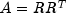, where R is a lower triangular matrix. Thus,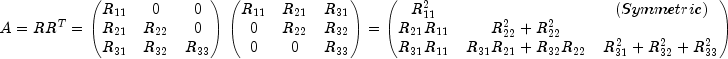which leads to the following for the entries of the lower triangular marix R: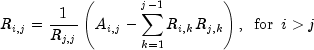and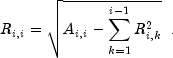The method `update` is based on the LINPACK routine `SCHUD`; see Dongarra et al. (1979) and updates the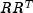Cholesky factorization of the real symmetric positive definite matrix A after a rank-one matrix is added. Given this factorization, `update` computes the factorization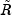such that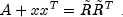Similarly, the method `downdate`, based on the LINPACK routine `SCHDD`; see Dongarra et al. (1979), downdates theCholesky factorization of the real symmetric positive definite matrix A after a rank-one matrix is subtracted. `downdate` computes the factorizationsuch that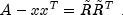This is not always possible, since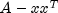may not be positive definite.

`downdate` determines an orthogonal matrix U as the product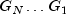of Givens rotations, such that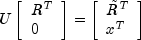By multiplying this equation by its transpose and noting that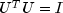, the desired result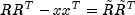is obtained.

Let a be the solution of the linear system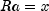and let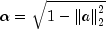The Givens rotations,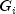, are chosen such that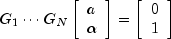The, are (N + 1) by (N + 1) matrices of the form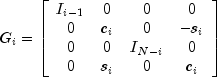where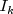is the identity matrix of order k; and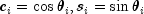for some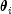.

The Givens rotations are then used to form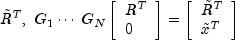The matrixis lower triangular and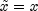because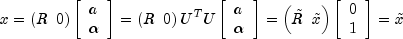.

Example, Serialized Form
• ### Nested Class Summary

Nested Classes
Modifier and Type Class and Description
`static class ` `Cholesky.NotSPDException`
The matrix is not symmetric, positive definite.
• ### Constructor Summary

Constructors
Constructor and Description
`Cholesky(double[][] a)`
Create the Cholesky factorization of a symmetric positive definite matrix of type `double`.
• ### Method Summary

Methods
Modifier and Type Method and Description
`void` `downdate(double[] x)`
Downdates the factorization by subtracting a rank-1 matrix.
`double[][]` `getR()`
Returns the R matrix that results from the Cholesky factorization.
`double[][]` `inverse()`
Returns the inverse of this matrix
`double[]` `solve(double[] b)`
Solve Ax = b where A is a positive definite matrix with elements of type `double`.
`void` `update(double[] x)`
• ### Methods inherited from class java.lang.Object

`clone, equals, finalize, getClass, hashCode, notify, notifyAll, toString, wait, wait, wait`
• ### Constructor Detail

• #### Cholesky

```public Cholesky(double[][] a)
throws SingularMatrixException,
Cholesky.NotSPDException```
Create the Cholesky factorization of a symmetric positive definite matrix of type `double`.
Parameters:
`a` - a `double` square matrix to be factored
Throws:
`IllegalArgumentException` - Thrown when the row lengths of matrix a are not equal (for example, the matrix edges are "jagged".)
`SingularMatrixException` - Thrown when the input matrix A is singular.
`Cholesky.NotSPDException` - Thrown when the input matrix is not symmetric, positive definite.
• ### Method Detail

• #### downdate

```public void downdate(double[] x)
throws Cholesky.NotSPDException```
Downdates the factorization by subtracting a rank-1 matrix. The object will contain the Cholesky factorization of, where A is the previously factored matrix.
Parameters:
`x` - A `double` array which specifies the rank-1 matrix. `x` is not modified by this function.
Throws:
`Cholesky.NotSPDException` - ifis not symmetric positive-definite.
• #### getR

`public double[][] getR()`
Returns the R matrix that results from the Cholesky factorization.
Returns:
a `double` matrix which contains the lower triangular R matrix that results from the Cholesky factorization such that• #### inverse

`public double[][] inverse()`
Returns the inverse of this matrix
Returns:
a `double` matrix containing the inverse
• #### solve

`public double[] solve(double[] b)`
Solve Ax = b where A is a positive definite matrix with elements of type `double`.
Parameters:
`b` - a `double` array containing the right-hand side of the linear system
Returns:
a `double` array containing the solution to the system of linear equations
• #### update

`public void update(double[] x)`
Updates the factorization by adding a rank-1 matrix. The object will contain the Cholesky factorization of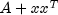, where A is the previously factored matrix.
Parameters:
`x` - A `double` array which specifies the rank-1 matrix. `x` is not modified by this function.
JMSLTM Numerical Library 7.2.0